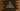# C++ program to find the first digit of the factorial of a number## C++ program to find the first digit of a factorial:

In this post, we will learn how to find the first digit of a factorial of a given number. The program will take the number as input from the user and print the first digit of the factorial.

### C++ program:

The easiest way to solve this problem is by calculating the factorial value and finding the first digit for that value. Factorial is the product of all numbers from 1 to that number. For a large number, it will cause overflow issue.

To make this problem more efficient, we will remove the last zeros of the number after each multiplication. It will not effect the final result because we need the first digit.

Below is the complete C++ program that solves this problem:

``````#include <iostream>
using namespace std;

int findFirstDigit(int number)
{
long long int factorial = 1;

for (int i = 2; i <= number; i++)
{
factorial *= i;

while (factorial % 10 == 0)
{
factorial = factorial / 10;
}
}

while (factorial > 10)
{
factorial = factorial / 10;
}

return factorial;
}

int main()
{
int number;
cout << "Enter a number : " << endl;
cin >> number;

cout << "First digit of factorial : " << findFirstDigit(number) << endl;

return 0;
}``````

### Explanation :

1. In this program, we are reading the number as user input and storing it in the variable number.
2. findFirstDigit method takes the user input value and returns the first digit of the factorial
3. It calculates the factorial of the given number by running a for loop that runs from i = 2 to that number. Inside the loop, it multiplies the current value of factorial with i. Then we are using a while loop to remove the last zeros from the result.
4. Once the loop ends, we are using another while loop to get the first digit from the factorial value.

### Sample Output:

``````Enter a number :
10
First digit of factorial : 3

Enter a number :
20
First digit of factorial : 2``````